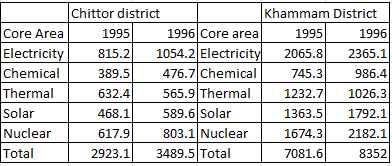### CAT 1996 Question Paper Question 61

Instructions

Answer the questions based on the following information. The amount of money invested (rupees in crores) in the core infrastructure areas of two districts, Chittoor and Khammam, Andhra Pradesh, is as follows.Question 61

# By what per cent was the total investment in the two districts more in 1996 as compared to 1995?

Solution

Total investment in 1996 = 3489.5 + 8352 = 11841.5
Total investment in 1995 = 7081.6 + 2923.1 = 10004.7
change = 1836.8
%change = $$\frac{1836.8}{10004.7} \times 100$$
As we can see with apprximation method that above value will be less than 20 and more than 18
Hence most appropriate answer will be D) = 18

• All Quant CAT Formulas and shortcuts PDF
• 30+ CAT previous papers with solutions PDF